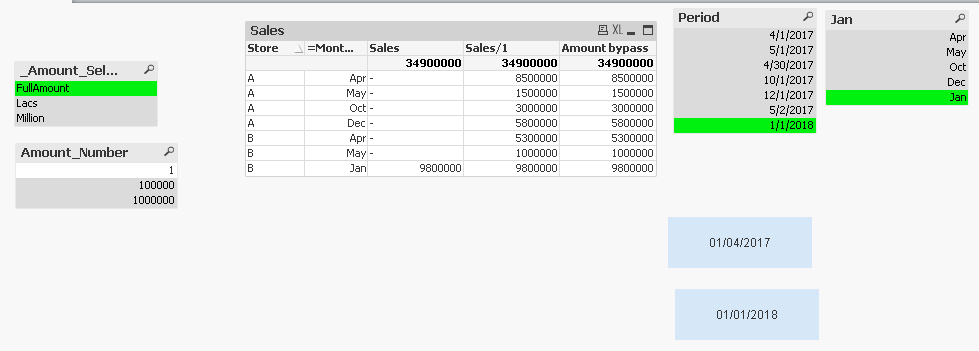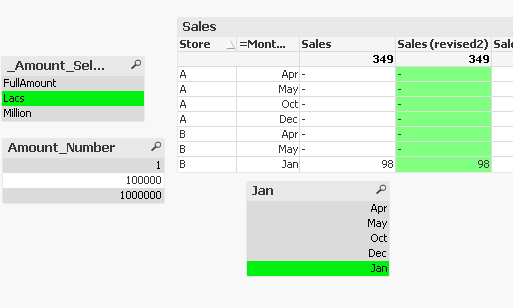# New to QlikView

Discussion board where members can get started with QlikView.

Announcements
Talk to Experts Tuesday, Live Q&A, September 22: Moving from QlikView to Qlik Sense. REGISTER
cancel
Showing results for
Did you mean:
HighlightedPartner

## Amount Conversion Issue

Dear All,

I have a strange issue on amount conversion like ! ! FullAmount,Lacs etc.

Lets check below scenario.

I have inline table for amount conversion.

AmountSelection:

FullAmount,1

Lacs,100000

Million,1000000

];

And lets take some data.

Store, Period, Sales

A, 4/1/2017, 2000000

A, 5/1/2017, 1500000

A, 4/30/2017, 6500000

A, 10/1/2017, 3000000

A, 12/1/2017, 5800000

B, 5/2/2017, 1000000

B, 4/30/2017, 5300000

B, 1/1/2018, 9800000

];

now I have this chart as follows-in First Column I have sales amount from 1-4-2017 to 1/1/2018

=sum({<Period={">=\$(vFinStart)<=\$(vMonthEnd)"},Period=>}Sales)/Amount_Number

this means It will show each month sale amount in fullamount,Lacs etc.

But In this case Total sale matching, but except last row all month data showing null.

Second column:  if I write sale amount/1 this is working. But I can not use this I need flexible selection of Amount.

=sum({<Period={">=\$(vFinStart)<=\$(vMonthEnd)"},Period=>}Sales)/1

Third Column: If I write sum(sale amount)/sum({<Period=>}Amount_Number) this is working.

=sum({<Period={">=\$(vFinStart)<=\$(vMonthEnd)"},Period=>}Sales)/sum({<Period=>}Amount_Number)

But I have 200 Reports I can't change the expression in all reports.

Is there any way to resolve this??

1 Solution

Accepted Solutions
HighlightedPartner

Hi Prabir,

You can also try this attached solution.

10 Replies
HighlightedPartner

Hi Prabir,

i got your point, rather than using as it is field create one variable

V Number=

=if(GetSelectedCount([Amount_Number])>1 or GetSelectedCount([Amount_Number])<1, 1,

if(GetFieldSelections([Amount_Number])='Lakh',100000,

if(GetFieldSelections([Amount_Number])='Fullamount',1,

if(GetFieldSelections([Amount_Number])='Million',1000000)

)

)

)

And in Expression simply use:

=sum({<Period={">=\$(vFinStart)<=\$(vMonthEnd)"},Period=>}Sales)/\$(vNumber)

Thanks,

Arvind Patil

HighlightedMVP

=Sum({<Period={">=\$(vFinStart)<=\$(vMonthEnd)"},Period=>}Sales/Amount_Number)

HighlightedPartner

Hello Arvind,

I have checked your resolution. it's working fine but as I have a large application in which multiple reports within 20 tabs. So, I can't change each & every report expression. Is there any way to do the same in script?

It will be a great help!

HighlightedSpecialist II

Hi Prabir

There are a few things that need to be fixed within your model; I don't think you can achieve the desired output without changes to the expression as well as to how the two variables are set.

Let's start with the variables; in your application you format the two date variables as DD/MM/YYYY (vMonthEnd=Date(Max(Period),'DD/MM/YYYY')). The issue is that your date format in the application is different (i.e. MM/DD/YYYY); so, your selections are not working with the set analysis. Also, in your set analysis you set the Period twice: {<Period={">=\$(vFinStart)<=\$(vMonthEnd)"},Period=>}. this means that Period is always ignored (i.e. "Period=") and the variables are never working.

Moving on to the expression; apart from the issue with the variables, the amount you are dividing by needs to be defined within the same data set. If you apply a set analysis to part of the expression but not to all of it some of the values will return null because there is no data for those dimensions (again, because you have not applied set analysis consistently in your expression). See "Table box - no set analysis" to understand what is the impact to the default state when you are making selections.

I've corrected the variable definitions and the formulas in your chart to show how I believe this should be implemented; see file test.qvw

The other option you might have is to separate the selection of the Period from the default state. Please see file test2.qvw. In this scenario I've created another table in the load script (_Periods) where I just load the dates. I have then linked the two variables and the list box to this new table. So, making dates selection won't impact the default state. You still have the issue with the set analysis where you define period twice( {<Period={">=\$(vFinStart)<=\$(vMonthEnd)"},Period=>}); I don't think you can avoid fixing it

HighlightedPartner

Hi Lorenzo,

Yes, You are right, I have a issue in my expression, {<Period={">=\$(vFinStart)<=\$(vMonthEnd)"},Period=>}.

I have removed it, But till now it's not working as per your test2.qvw app.And as per your test.qvw app you have write

=sum({<Period={">=\$(vFinStart)<=\$(vMonthEnd)"}>}Sales)/only({<Period=>}Amount_Number)

It's working, but i have to change this in all reports , all expression!!!!

HighlightedMVP

Is this something you can do?

=Sum({<Period={">=\$(vFinStart)<=\$(vMonthEnd)"},Period=>}Sales/Amount_Number)

HighlightedPartner

Hi Sunny,

Actually, I have to use this expression:

=Sum({<Period={">=\$(vFinStart)<=\$(vMonthEnd)"}>}Sales/Amount_Number)

But I have to Change in All Expression..

HighlightedSpecialist II

See attached test2.qvw file. I've updated the expressions behind the month selection

Also, I've amended test.qvw following Sunny's feedback above

I don't think you can get away without fixing the expressions

HighlightedPartner

Hi Prabir,

You can also try this attached solution.